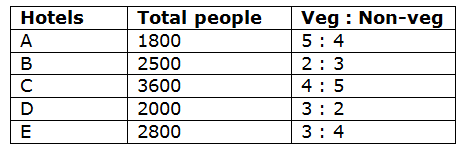# SBI Clerk Prelims 2020 Quantitative Aptitude Questions (Day-15)

Dear Aspirants, Our IBPS Guide team is providing new series of Quantitative Aptitude Questions for SBI Clerk Prelims 2020 so the aspirants can practice it on a daily basis. These questions are framed by our skilled experts after understanding your needs thoroughly. Aspirants can practice these new series questions daily to familiarize with the exact exam pattern and make your preparation effective.

[WpProQuiz 7659]

Ensure Your Ability Before the Exam – Take SBI Clerk 2020 Prelims Free Mock Test

Simplification

Directions (Q. 1 – 5): What value should come in place of question mark (?) in the following questions?

1) √16384 ÷ 4 × 16 = ? – 18312 ÷ 12 + 142

a) 1678

b) 1842

c) 1426

d) 2154

e) None of these

2) (7/9) of 4068 + 77 % of 1200 = (?)2 – (128 ÷ 16)

a) 64

b) 62

c) 58

d) 56

e) None of these

3) (8 × 8)5 ÷ (512 ÷ 8)2 × (8 × 64)3 = (8)? + 5

a) 12

b) 8

c) 6

d) 10

e) None of these

4) ? % of (75 × 4 – 150) = 420 + 752

a) 4280

b) 4520

c) 4030

d) 5060

e) None of these

5) (7/84) of (12/156) of 4680 = 45 – ?

a) 15

b) 20

c) 25

d) 30

e) None of these

Data Interpretation

Directions (Q. 6 – 10): Study the following information carefully and answer the given questions?

The following table shows the total number of people (Male + Female) went for 5 different hotels and ate some veg and non-veg items in a certain week. The ratio of veg to that of non-veg items also given here.6) Find the difference between the total number of male eating veg to that of total number of female eating non-veg from hotel A, if the percentage of total number of female eating veg and that of male eating non-veg from hotel A is 52 % and 45 %?

a) 40

b) 60

c) 80

d) 20

e) None of these

7) Find the ratio between the total number of people eating Veg from hotel B and D together to that of total number of people eating non-veg from hotel C and E together?

a) 23 : 29

b) 19 : 25

c) 34 : 47

d) 11 : 18

e) None of these

8) Find the total number of people eating non-veg from hotel B, C and D together?

a) 3800

b) 4300

c) 4700

d) 3500

e) None of these

9) Total number of people eating non-veg from hotel E is what percentage of total number of people eating veg from hotel A?

a) 125 %

b) 140 %

c) 160 %

d) 175 %

e) None of these

10) Find the difference between the average number of people went for hotel A and C together to that of the average number of people went for hotel B and D together?

a) 600

b) 300

c) 750

d) 450

e) None of these

Directions (1-5) :

√16384 ÷ 4 × 16 = x – 18312 ÷ 12 + 142

(128/4) * 16 = x – (18312 / 12) + 196

512 = x – 1526 + 196

x = 512 + 1526 – 196 = 1842

(7/9) * 4068 + (77/100) * 1200 = x2 – (128/16)

3164 + 924 + 8 = x2

x2 = 4096

x = 64

(82)5 ÷ (512/8)2 × (8 × 82)3 = (8)x + 5

810 ÷ (64)2 × (83)3 = (8)x + 5

810 ÷ 84 × 89 = (8)x + 5

810 – 4 + 9 = 8x + 5

815 = 8x + 5

15 = x + 5

x = 10

x % of (75 × 4 – 150) = 420 + 752

(x/100) * (300 – 150) = 420 + 5625

(x/100) * 150 = 6045

3x/2 = 6045

x = 6045 * (2/3) = 4030

(7/84) * (12/156) * 4680 = 45 – x

30 = 45 – x

x = 45 – 30 = 15

Directions (6-10) :

The total number of male eating veg from hotel A

= > 1800 * (5/9) * (48/100) = 480

The total number of female eating non-veg from hotel A

= > 1800 * (4/9) * (55/100) = 440

Required difference = 480 – 440 = 40

The total number of people eating Veg from hotel B and D together

= > 2500 * (2/5) + 2000 * (3/5)

= > 1000 + 1200 = 2200

The total number of people eating non-veg from hotel C and E together

= > 3600 * (5/9) + 2800 * (4/7)

= > 2000 + 1600 = 3600

Required ratio = 2200 : 3600 = 11 : 18

The total number of people eating non-veg from hotel B, C and D together

= > [2500 * (3/5) + 3600 * (5/9) + 2000 * (2/5)]

= > [1500 + 2000 + 800] = 4300

Total number of people eating non-veg from hotel E

= > 2800 * (4/7) = 1600

Total number of people eating Veg from hotel A

= > 1800 * (5/9) = 1000

Required % = (1600 / 1000) * 100 = 160 %

The average number of people went for hotel A and C together

= > (1800 + 3600) / 2 = 2700

The average number of people went for hotel B and D together

= > (2500 + 2000) / 2 = 2250

Required difference = 2700 – 2250 = 450

 Check Here to View SBI Clerk Prelims 2020 Quantitative Aptitude Questions Day 14 Day 13 Day 12 Click Here for SBI Clerk 2020 – Detailed Exam Notification